Next: Heisenberg's Uncertainty Principle Up: Wave Mechanics Previous: Probability Interpretation of Wavefunction

# Wave Packets

As we have seen, the wavefunction of a massive particle of momentum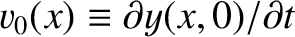and energy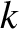, moving in free space along the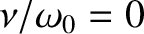-axis, can be written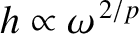(1116)

where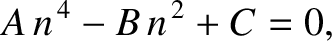,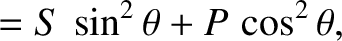, and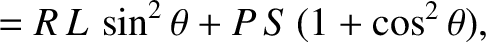is a complex constant. Here,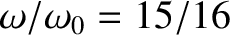andare linked via the matter wave dispersion relation (1103). Expression (1116) represents a plane wave that propagates in the-direction with the phase velocity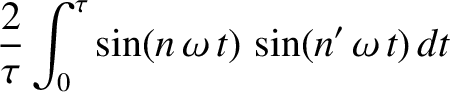. However, it follows from Equation (1104) that this phase velocity is only half of the classical velocity of a massive particle.

According to the discussion in the previous section, the most reasonable physical interpretation of the wavefunction is that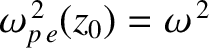is proportional to (assuming that the wavefunction is not properly normalized) the probability of finding the particle betweenand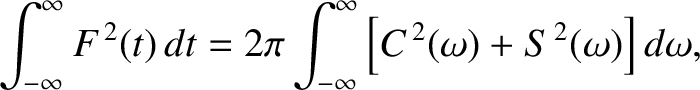at time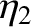. However, the modulus squared of the wavefunction (1116) is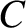, which is a constant that depends on neithernor. In other words, the previous wavefunction represents a particle that is equally likely to be found anywhere on the-axis at all times. Hence, the fact that this wavefunction propagates at a phase velocity that does not correspond to the classical particle velocity has no observable consequences.

How can we write the wavefunction of a particle that is localized in? In other words, a particle that is more likely to be found at some positions on the-axis than at others. It turns out that we can achieve this goal by forming a linear combination of plane waves of different wavenumbers: that is,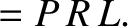(1117)

Here,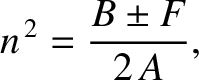represents the complex amplitude of plane waves of wavenumberwithin this combination. In writing the previous expression, we are relying on the assumption that matter waves are superposable. In other words, it is possible to add two valid wave solutions to form a third valid wave solution. The ultimate justification for this assumption is that matter waves satisfy the linear wave equation (1102).

There is a fundamental mathematical theorem, known as Fourier's theorem (see Section 9.1 and Exercise 11), which states that if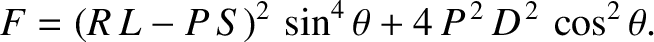(1118)

then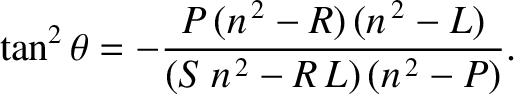(1119)

Here,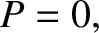is known as the Fourier transform of the function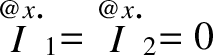. We can use Fourier's theorem to find the-space functionthat generates any given-space wavefunction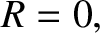at a given time.

For instance, suppose that atthe wavefunction of our particle takes the form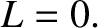(1120)

Thus, the initial probability distribution for the particle's-coordinate is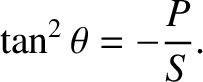(1121)

This particular distribution is called a Gaussian distribution (see Section 9.1), and is plotted in Figure 79. It can be seen that a measurement of the particle's position is most likely to yield the value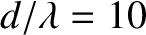, and very unlikely to yield a value which differs fromby more than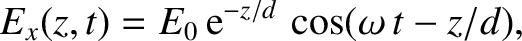. Thus, Equation (1120) is the wavefunction of a particle that is initially localized in some region of-space, centered on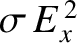, whose width is of order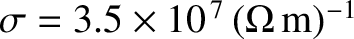. This type of wavefunction is known as a wave packet. However, a wave packet is just another name for a wave pulse. (See Chapter 9.)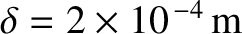According to Equation (1117),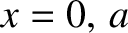(1122)

Hence, we can employ Fourier's theorem to invert this expression to give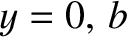(1123)

Making use of Equation (1120), we obtain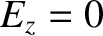(1124)

Changing the variable of integration to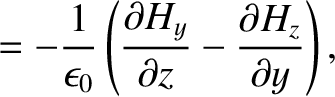, the previous expression reduces to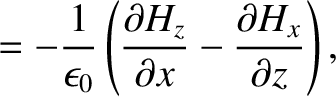(1125)

whereand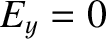. The integral in the previous equation is now just a number, as can easily be seen by making the second change of variable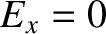. Hence, we deduce that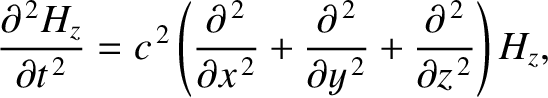(1126)

where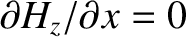(1127)

If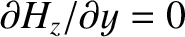is proportional to the probability of a measurement of the particle's position yielding a value in the rangetoat timethen it stands to reason that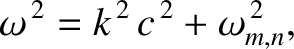is proportional to the probability of a measurement of the particle's wavenumber yielding a value in the rangeto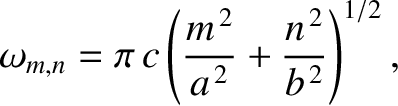. (Recall that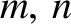, so a measurement of the particle's wavenumber,, is equivalent to a measurement of the particle's momentum,). According to Equation (1126),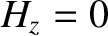(1128)

This probability distribution is a Gaussian in-space. [See Equation (1121) and Figure 79.] Hence, a measurement ofis most likely to yield the value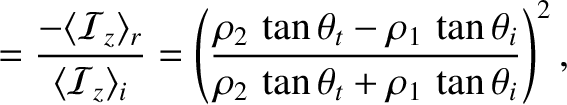, and very unlikely to yield a value that differs fromby more than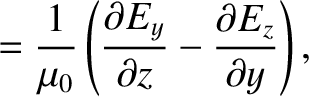.

We have just seen that a wave packet with a Gaussian probability distribution of characteristic widthin-space [see Equation (1121)] is equivalent to a wave packet with a Gaussian probability distribution of characteristic width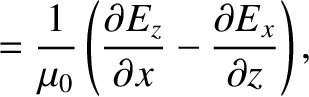in-space [see Equation (1128)], where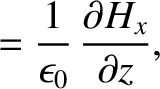(1129)

This illustrates an important property of wave packets. Namely, in order to construct a packet that is highly localized in-space (i.e., with small) we need to combine plane waves with a very wide range of different-values (i.e., with large). Conversely, if we only combine plane waves whose wavenumbers differ by a small amount (i.e., ifis small) then the resulting wave packet is highly extended in-space (i.e.,is large).

According to Section 10.1, a wave packet made up of a superposition of plane waves that is strongly peaked around some central wavenumberpropagates at the group velocity,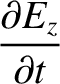(1130)

rather than the phase velocity,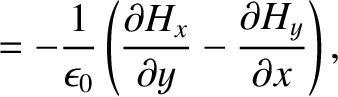, assuming that all of the constituent plane waves satisfy a dispersion relation of the form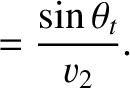. For the case of matter waves, the dispersion relation is (1103). Thus, the associated group velocity is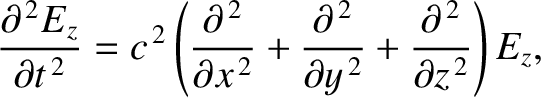(1131)

where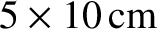. This velocity is identical to the classical velocity of a (non-relativistic) massive particle. We conclude that the matter wave dispersion relation (1103) is perfectly consistent with classical physics, as long as we recognize that particles must be identified with wave packets (which propagate at the group velocity) rather than plane waves (which propagate at the phase velocity).

In Section 10.1, it was demonstrated that the spatial extent of a wave packet of initial extent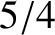grows, as the packet evolves in time, like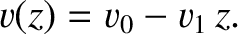(1132)

whereis the packet's central wavenumber. Thus, it follows from the matter wave dispersion relation, (1103), that the width of a particle wave packet grows in time as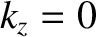(1133)

For example, if an electron wave packet is initially localized in a region of atomic dimensions (i.e.,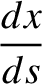) then the width of the packet doubles in about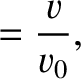.Next: Heisenberg's Uncertainty Principle Up: Wave Mechanics Previous: Probability Interpretation of Wavefunction
Richard Fitzpatrick 2013-04-08